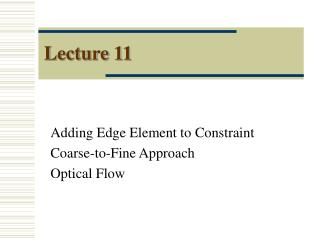DownloadDownload PresentationLecture 11

# Lecture 11

Download Presentation## Lecture 11

- - - - - - - - - - - - - - - - - - - - - - - - - - - E N D - - - - - - - - - - - - - - - - - - - - - - - - - - -
##### Presentation Transcript

1. Lecture 11 Adding Edge Element to Constraint Coarse-to-Fine Approach Optical Flow

2. Edge Constraint for Noise Removal Vertical Edge Element ON/OFF Horizontal Edge Element ON/OFF Tends to create problems at edges If edge element “ON”, turn off smoothness

3. Edge Constraint for Noise Removal Cost3 = 0 Cost3 = 1 Cost3 = 0.1

4. Edge Shape Precedences Vij Dij Hij Hij Vij Dij

5. Algorithm 1. Start Temperature T is high 2. Initialize D(i,j) = Random 0..10 3. For each pixel (i,j) if Dij = 0,Vij = 0, Hij = 0; E0= ... ; P0 = e - E0 /T if Dij = 0,Vij = 0, Hij = 1; E1 = ... ; P1 = e - E1 /T . . if Dij = 10,Vij = 1, Hij = 1; E44 = ... ; P44 = e – E44 /T For each Pi = Pi/Z Pi/sum(Pi) Sample for state K from pdf Pi Dij = 1,Vij = 0, Hij = 0 4. Reduce T 5. Repeat step 3-4 while E is not stable

6. Coarse-to-Fine 101 Right Left D Fine Dm = 20 Dhalf Dm1/2 = 10 41 D1/4 Dm1/4 = 5 Solve First Coarse 2

7. Coarse-to-Fine - Pyramid

8. Same image Plane One Point Stereo – Parallel Axis Matching Points will be in the same row

9. Stereo – Parallel Axis Epipolar – Line formed by intersection of epipolar plane with image plane Epipolar Plane – Planes defined by 2 camera center and viewed 3-D point

10. Motion – Optical Flow Movement of Image Points Frame 2 Frame 1 Optical Flow is written as (u,v)

11. Motion Correspondence Problem 1. Start Temperature T is high 2. u = Random (-5,-4,….,0,…., 4, 5); v = Random (-5,-4,….,0,…., 4, 5) 3. For every (edge) pixel if u = -5, v = -5; E0= ... ; P0 = e - E0 /T if u = -5, v = -4; E1 = ... ; P1 = e - E1 /T . . if u = 5, v = 5; E121 = ... ; P121 = e – E121 /T For each Pi = Pi/Z Pi/sum(Pi) Sample for (u,v) from pdf Pi 4. Reduce T 5. Repeat step 3-4 while E is not stable

12. Stereo Matching : Disparity Can add 1-1 match constraint

13. Barber Pole Illusion Aperture Problem – Perceive that motion is perpendicular to edge